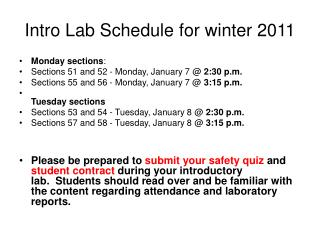DownloadDownload PresentationIntro Lab Schedule for winter 2011

# Intro Lab Schedule for winter 2011

Télécharger la présentation## Intro Lab Schedule for winter 2011

- - - - - - - - - - - - - - - - - - - - - - - - - - - E N D - - - - - - - - - - - - - - - - - - - - - - - - - - -
##### Presentation Transcript

1. Intro Lab Schedule for winter 2011 • Monday sections: • Sections 51 and 52 - Monday, January 7 @ 2:30 p.m. • Sections 55 and 56 - Monday, January 7 @ 3:15 p.m. • Tuesday sections • Sections 53 and 54 - Tuesday, January 8 @ 2:30 p.m. • Sections 57 and 58 - Tuesday, January 8 @ 3:15 p.m. • Please be prepared to submit your safety quiz and student contract during your introductory lab.  Students should read over and be familiar with the content regarding attendance and laboratory reports.

2. Chapter 7: Chemical Equilibrium

3. 7.1 The Gibbs energy minimum 1. Extent of reaction (ξ): The amount of reactants being converted to products. Its unit is mole. Consider: A ↔ B Assume an infinitesimal amount dξ of A turns into B, dnA = -dξ On the other hand, dnB = dξ • Why do we need a new quantity? consider a generic reaction: 2A ↔ 3B • In a very general way, the extent of reaction is calculated as dξ = dnA/vA (where vA is the stoichiometric number of the reactant A, which is negative for the reactant!!)

4. Example 1: N2(g) + 3H2(g) ↔ 2NH3(g) when the extent of reaction changes from ξ = 0 to ξ = 1.0 mole, what are the changes of each reagent? Solution: identify vj: v(N2) = -1; v(H2) = -3; v(NH3) = 2. since dξ = 1.0 mole, dn(N2) = -1x1.0 mole = -1.0 mole, dn(H2) = -3x1.0 mole = -3.0 moles, dn(NH3) = 2x1.0 mole = 2.0 moles, Example 2: CH4(g) + Cl2(g) ↔ CHCl3(l) + HCl(g), in which the amount of reactant Cl2(g) decreases by 2 moles. What is the extent of the reaction? Solution:

5. Variation of Gibbs energy during a reaction process

6. The Reaction Gibbs energy: ΔrG the slope of the Gibbs energy plotted against the extent of reaction: ΔrG = here Δr signifies a derivative • A reaction for which ΔrG < 0 is called exergonic. • A reaction for which ΔrG > 0 is called endergonic. • ΔrG < 0, the forward reaction is spontaneous. • ΔrG > 0, the reverse reaction is spontaneous. • ΔrG = 0, the reaction is at equilibrium!!!

7. An useful description of the reaction Gibbs energy (ΔrG) • Consider the reaction A ↔ B initial amount nA0 nB0 final amount nAf nBf Ginitial = uBnB0 + uAnA0 Gfinal = uBnBf + uAnAf ΔG = Gfinal - Ginitial = (uBnBf + uAnAf ) – (uBnB0 + uAnA0 ) = uB(nBf- nB0) + uA (nAf - nA0) = uBΔξ + uA(-Δξ) = (uB - uA )Δξ ΔrG = = uB - uA When uA > uB, the reaction A → B is spontaneous. When uB > uA, the reverse reaction (B → A) is spontaneous. When uB = uA, the reaction is spontaneous in neither direction (equilibrium condition).

8. 7.2 The description of equilibrium 1. Perfect gas equilibrium: A(g)↔ B(g) ΔrG = uB – uA

9. At equilibrium, ΔrG = 0, therefore Note: The difference in standard molar Gibbs energies of the products and reactants is equal to the difference in their standard Gibbs energies of formation, thus, ΔrGθ = ΔfGθ(B) - ΔfGθ(A)

10. Equilibrium for a general reaction Example: 2A + B ↔ C + 3D • The reaction Gibbs energy, ΔrG, is defined in the same way as discussed earlier: where the reaction quotient, Q, has the form: Q = activities of products/activities of reactants in a compact expression Q = Π αjνj vj are the corresponding stoichiometric numbers; positive for products and negative for reactants. vA = -2; vB = -1; vC = 1; vD = 3

11. Justification of the equation dG = ∑ujdnj Assuming that the extent of reaction equals dξ, one gets dnj = vjdξ then dG = ∑ujdnj = ∑ujvjdξ (dG/dξ) = ∑ujvj ΔrG = ∑ujvj because uj = ujθ + RTln(aj) ΔrG = ∑{vj(ujθ + RTln(aj))} = ∑(vjujθ) + ∑vj(RTln(aj)) = ΔrGθ + RT ∑ln(aj)vj = ΔrGθ + RT ln (∏(aj)vj) = ΔrGθ + RT ln (Q)

12. Again, we use K to denote the reaction quotient at the equilibrium point, K = Qequilibrium = (∏ajvj)equilibrium K is called thermodynamic equilibrium constant. Note that until now K is expressed in terms of activities) Example: calculate the quotient for the reaction: A + 2B ↔ 3C + 4D Solution: first, identify the stoichiometric number of each reactant: vA = -1, vB = -2, vC = 3, and vD = 4. At the equilibrium condition:

13. Examples of calculating equilibrium constants • Consider a hypothetical equilibrium reaction A(g) + B(g) ↔ C(g) + D(g) While all gases may be considered ideal. The following data are available for this reaction: Compound μθ(kJ mol-1) A(g) -55.00 B(g) -44.00 C(g) -54.00 D(g) -47.00 Calculate the value of the equilibrium constant Kp for the reaction at 298.15K. Solution:

14. Example 2: Using the data provided in the data section, calculate the standard Gibbs energy and the equilibrium constant at 25oC for the following reaction CH4(g) + Cl2(g) ↔ CHCl3(g) + HCl(g) Solution: (chalkboard) ΔfGθ(CHCl3, g) = -73.66 kJ mol-1 ΔfGθ(HCl, g) = - 95.30 kJ mol-1 ΔfGθ(CH4, g) = - 50.72 kJ mol-1